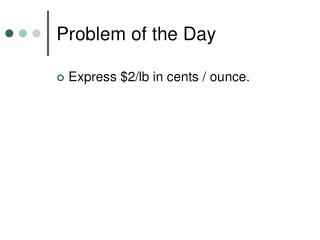DownloadDownload PresentationProblem of the Day

# Problem of the Day

Download Presentation## Problem of the Day

- - - - - - - - - - - - - - - - - - - - - - - - - - - E N D - - - - - - - - - - - - - - - - - - - - - - - - - - -
##### Presentation Transcript

1. Problem of the Day • Express \$2/lb in cents / ounce.

2. Ratio and Proportion A ratio is a comparison of two numbers by division. The ratio of a to b is a:b or a/b where b is not equal to zero.

3. Rate is a comparison of a/b where a and b represent two different units. • m/h, \$/h, \$/package • The unit rate is a a rate with a denominator of 1 • \$3.15/ 1 gallon • \$2.50/ 1 gallon

4. Find the unit rate of an ounce of catsup. • \$.72/16 ounces—Divide numerator and denominator by 16. • \$.045/ounce is the unit rate.

5. Converting rates • The boy ran 10,560 feet in 4 hours. What was his speed in feet per minute? • 10,560 feet/4 hr x 1hr/60min=176 feet/min

6. A proportion is an equation that states two ratios are equal. • t/9 = 5/6 is a proportion. To solve for t cross-multiply and divide. • 9 ∙ 5 = 6 ∙ t • 45 = 6 t • 45/6 = t • t = 7.5

7. You try it. • A sloth travels .15 miles per hour. Convert this speed to feet per minute. • Solve x/4 = 25/12 • 24/5 = y/7 • 54/d = 72/64

8. Homework • P. 185 and 186 (2-40even • )